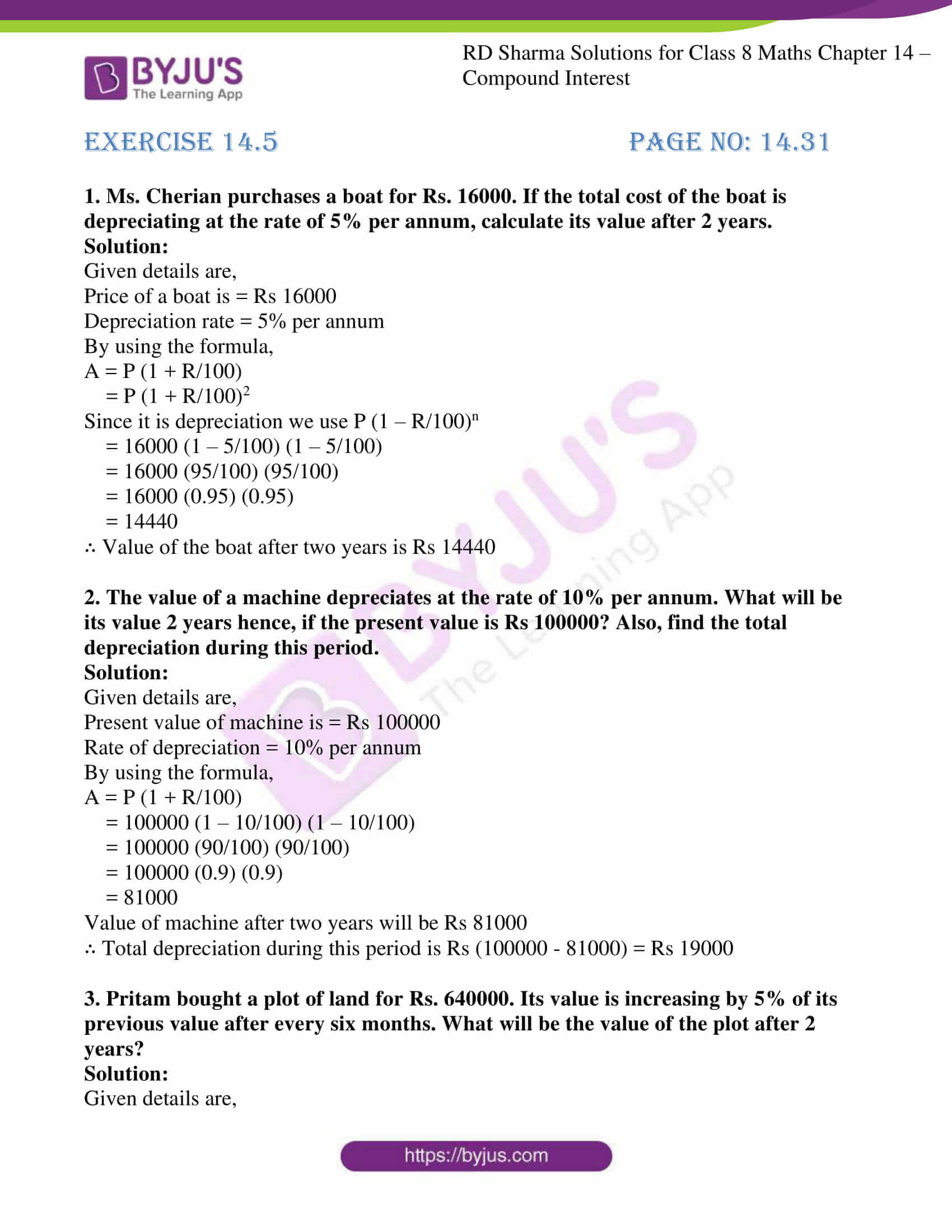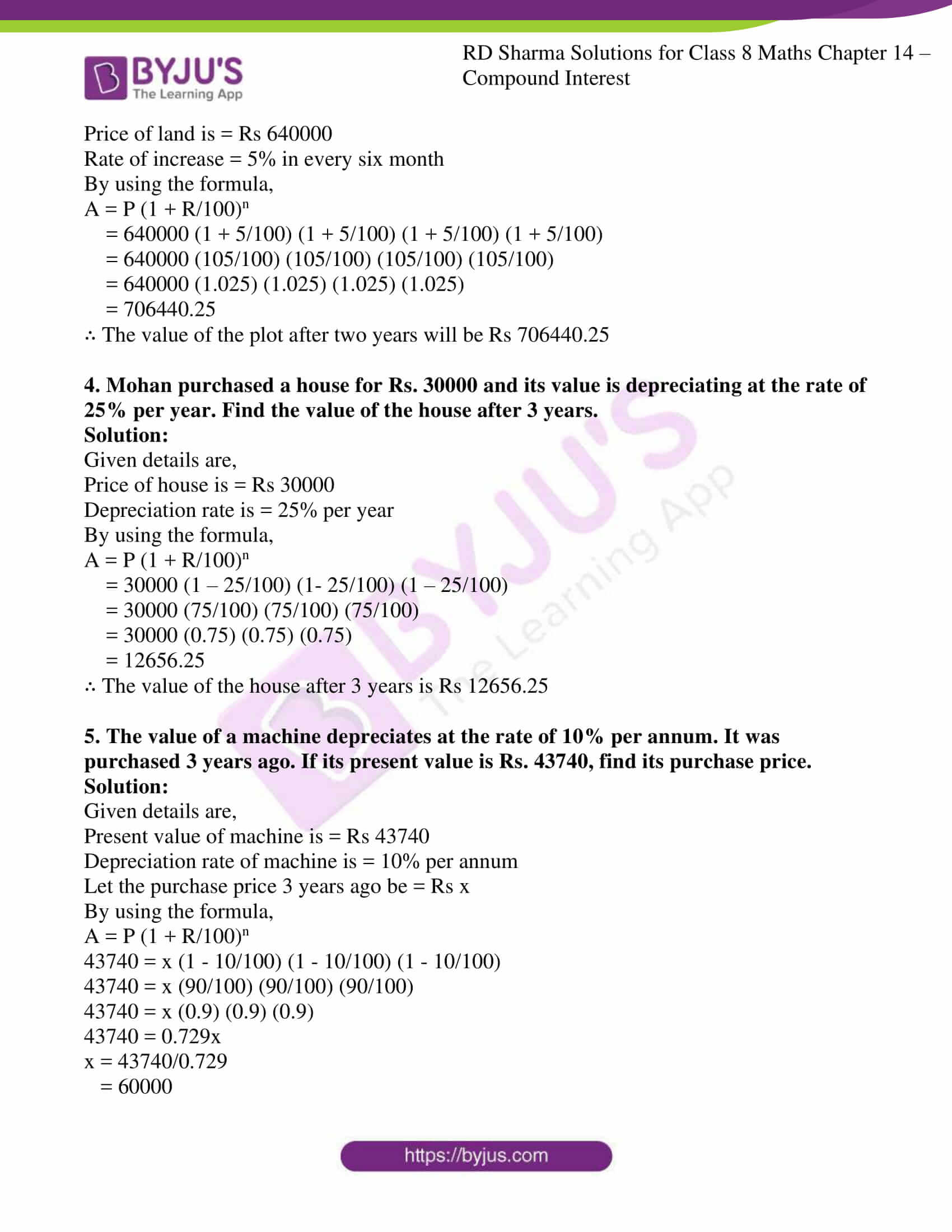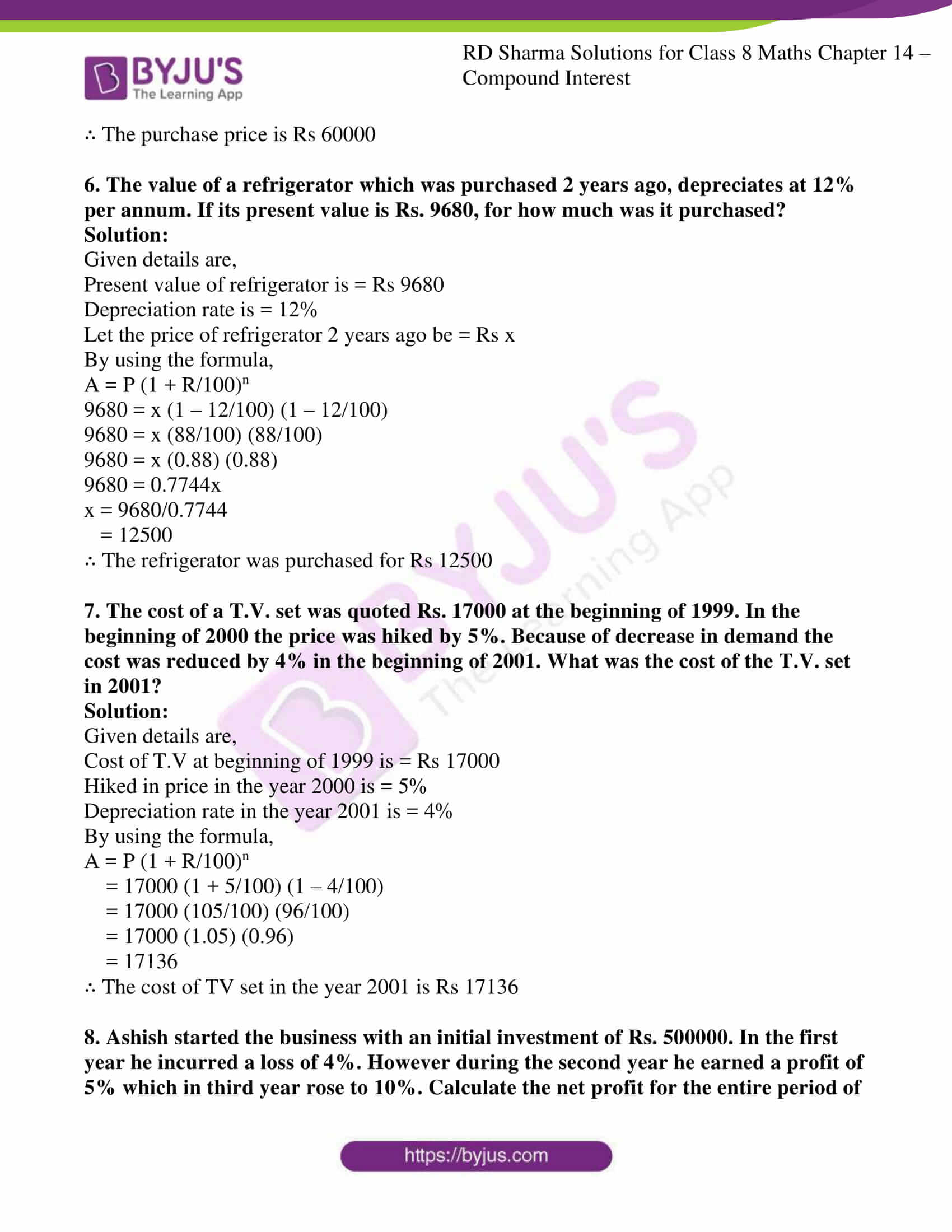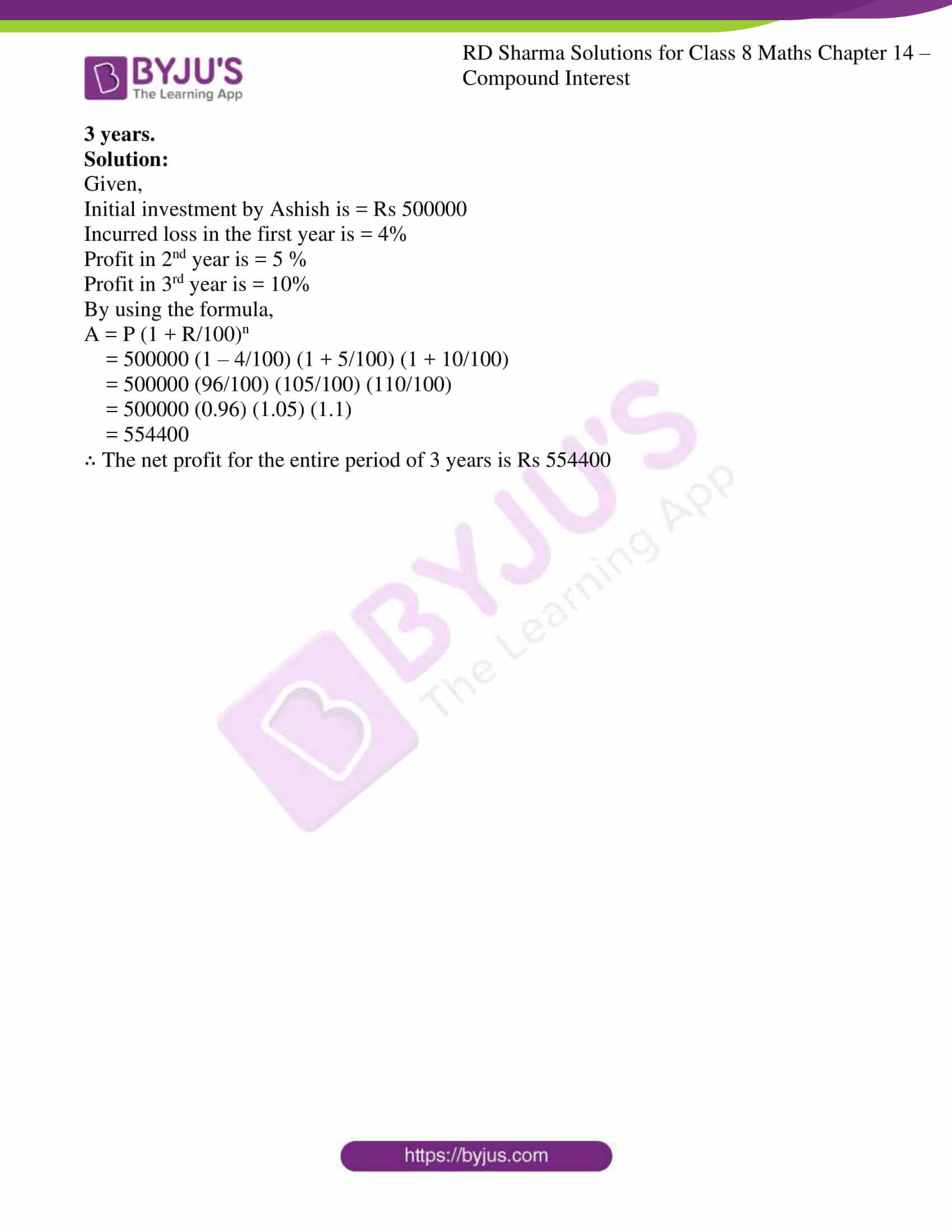# RD Sharma Solutions for Class 8 Maths Chapter 14 Compound Interest Exercise 14.5

We all know the fact that continuous use of any machine or article decreases in value with time. The relative decrease of any article over a period of time is called its depreciation. In Exercise 14.5 of Chapter 14, we shall study problems based on depreciation. Scoring good marks in the exam is the dream of any student. To achieve their dreams, students can refer to RD Sharma Solutions which is formulated in a step by step format by our experts at BYJU’S. To help students solve the problems at ease our tutors have provided detailed solutions to the problems. Pdfs can also be downloaded easily from the links given below.

## Download the Pdf of RD Sharma Solutions for Class 8 Maths Exercise 14.5 Chapter 14 Compound Interest### Access answers to Maths RD Sharma Solutions For Class 8 Exercise 14.5 Chapter 14 Compound Interest

1. Ms. Cherian purchases a boat for Rs. 16000. If the total cost of the boat is depreciating at the rate of 5% per annum, calculate its value after 2 years.

Solution:

Given details are,

Price of a boat is = Rs 16000

Depreciation rate = 5% per annum

By using the formula,

A = P (1 + R/100)

= P (1 + R/100)2

Since it is depreciation we use P (1 – R/100)n

= 16000 (1 – 5/100) (1 – 5/100)

= 16000 (95/100) (95/100)

= 16000 (0.95) (0.95)

= 14440

∴ Value of the boat after two years is Rs 14440

2. The value of a machine depreciates at the rate of 10% per annum. What will be its value 2 years hence, if the present value is Rs 100000? Also, find the total depreciation during this period.

Solution:

Given details are,

Present value of machine is = Rs 100000

Rate of depreciation = 10% per annum

By using the formula,

A = P (1 + R/100)

= 100000 (1 – 10/100) (1 – 10/100)

= 100000 (90/100) (90/100)

= 100000 (0.9) (0.9)

= 81000

Value of machine after two years will be Rs 81000

∴ Total depreciation during this period is Rs (100000 – 81000) = Rs 19000

3. Pritam bought a plot of land for Rs. 640000. Its value is increasing by 5% of its previous value after every six months. What will be the value of the plot after 2 years?

Solution:

Given details are,

Price of land is = Rs 640000

Rate of increase = 5% in every six month

By using the formula,

A = P (1 + R/100)n

= 640000 (1 + 5/100) (1 + 5/100) (1 + 5/100) (1 + 5/100)

= 640000 (105/100) (105/100) (105/100) (105/100)

= 640000 (1.025) (1.025) (1.025) (1.025)

= 706440.25

∴ The value of the plot after two years will be Rs 706440.25

4. Mohan purchased a house for Rs. 30000 and its value is depreciating at the rate of 25% per year. Find the value of the house after 3 years.

Solution:

Given details are,

Price of house is = Rs 30000

Depreciation rate is = 25% per year

By using the formula,

A = P (1 + R/100)n

= 30000 (1 – 25/100) (1- 25/100) (1 – 25/100)

= 30000 (75/100) (75/100) (75/100)

= 30000 (0.75) (0.75) (0.75)

= 12656.25

∴ The value of the house after 3 years is Rs 12656.25

5. The value of a machine depreciates at the rate of 10% per annum. It was purchased 3 years ago. If its present value is Rs. 43740, find its purchase price.

Solution:

Given details are,

Present value of machine is = Rs 43740

Depreciation rate of machine is = 10% per annum

Let the purchase price 3 years ago be = Rs x

By using the formula,

A = P (1 + R/100)n

43740 = x (1 – 10/100) (1 – 10/100) (1 – 10/100)

43740 = x (90/100) (90/100) (90/100)

43740 = x (0.9) (0.9) (0.9)

43740 = 0.729x

x = 43740/0.729

= 60000

∴ The purchase price is Rs 60000

6. The value of a refrigerator which was purchased 2 years ago, depreciates at 12% per annum. If its present value is Rs. 9680, for how much was it purchased?

Solution:

Given details are,

Present value of refrigerator is = Rs 9680

Depreciation rate is = 12%

Let the price of refrigerator 2 years ago be = Rs x

By using the formula,

A = P (1 + R/100)n

9680 = x (1 – 12/100) (1 – 12/100)

9680 = x (88/100) (88/100)

9680 = x (0.88) (0.88)

9680 = 0.7744x

x = 9680/0.7744

= 12500

∴ The refrigerator was purchased for Rs 12500

7. The cost of a T.V. set was quoted Rs. 17000 at the beginning of 1999. In the beginning of 2000 the price was hiked by 5%. Because of decrease in demand the cost was reduced by 4% in the beginning of 2001. What was the cost of the T.V. set in 2001?

Solution:

Given details are,

Cost of T.V at beginning of 1999 is = Rs 17000

Hiked in price in the year 2000 is = 5%

Depreciation rate in the year 2001 is = 4%

By using the formula,

A = P (1 + R/100)n

= 17000 (1 + 5/100) (1 – 4/100)

= 17000 (105/100) (96/100)

= 17000 (1.05) (0.96)

= 17136

∴ The cost of TV set in the year 2001 is Rs 17136

8. Ashish started the business with an initial investment of Rs. 500000. In the first year he incurred a loss of 4%. However during the second year he earned a profit of 5% which in third year rose to 10%. Calculate the net profit for the entire period of 3 years.

Solution:

Given,

Initial investment by Ashish is = Rs 500000

Incurred loss in the first year is = 4%

Profit in 2nd year is = 5 %

Profit in 3rd year is = 10%

By using the formula,

A = P (1 + R/100)n

= 500000 (1 – 4/100) (1 + 5/100) (1 + 10/100)

= 500000 (96/100) (105/100) (110/100)

= 500000 (0.96) (1.05) (1.1)

= 554400

∴ The net profit for the entire period of 3 years is Rs 554400